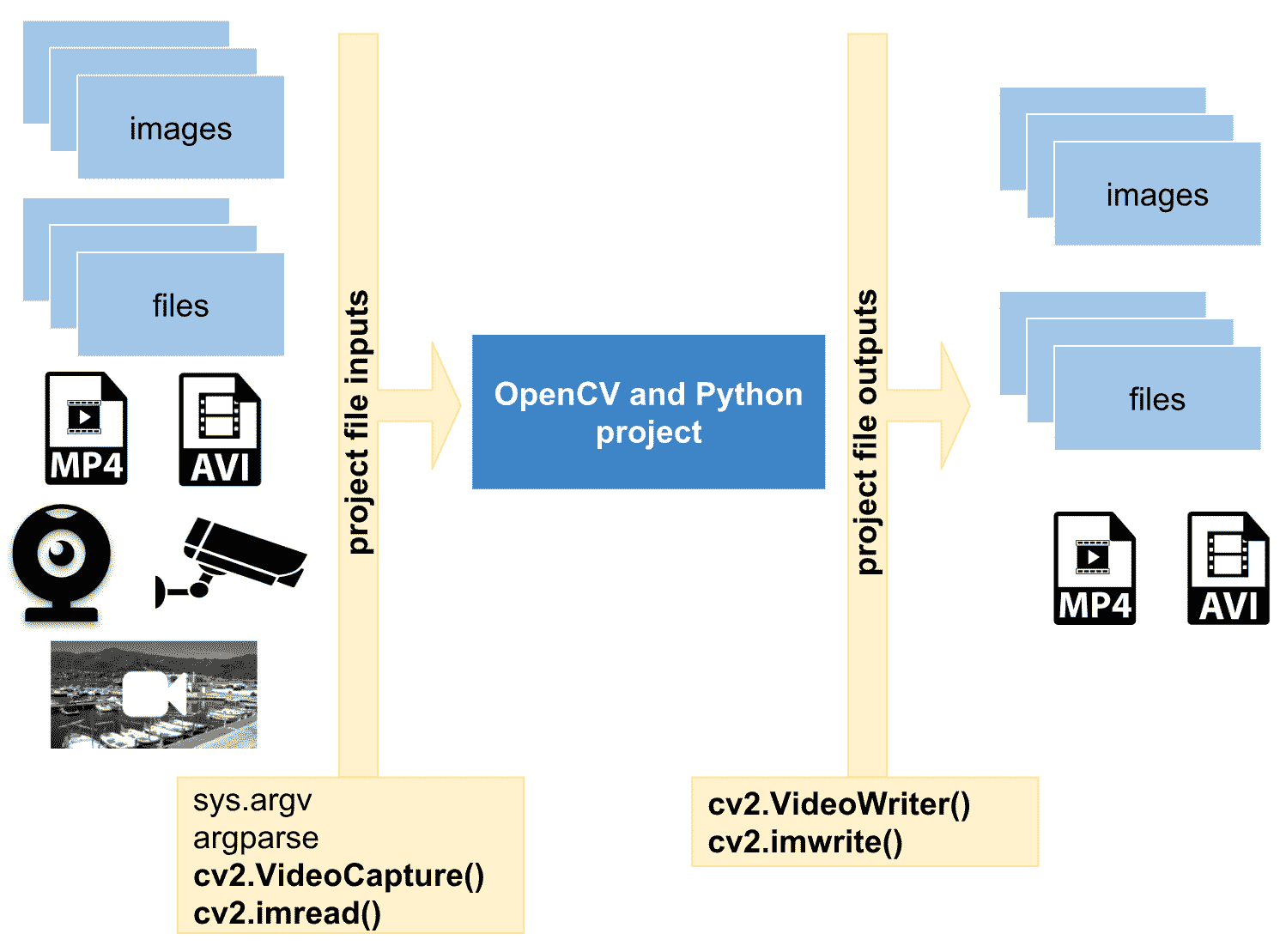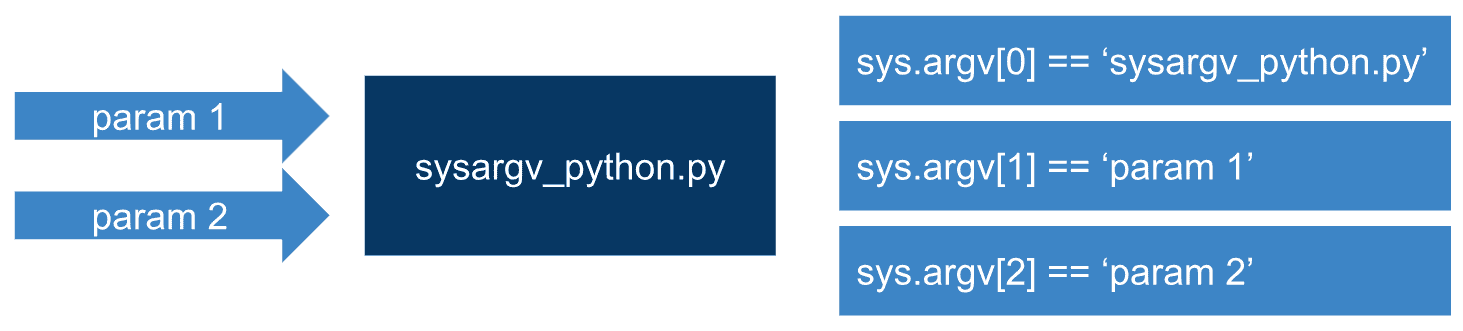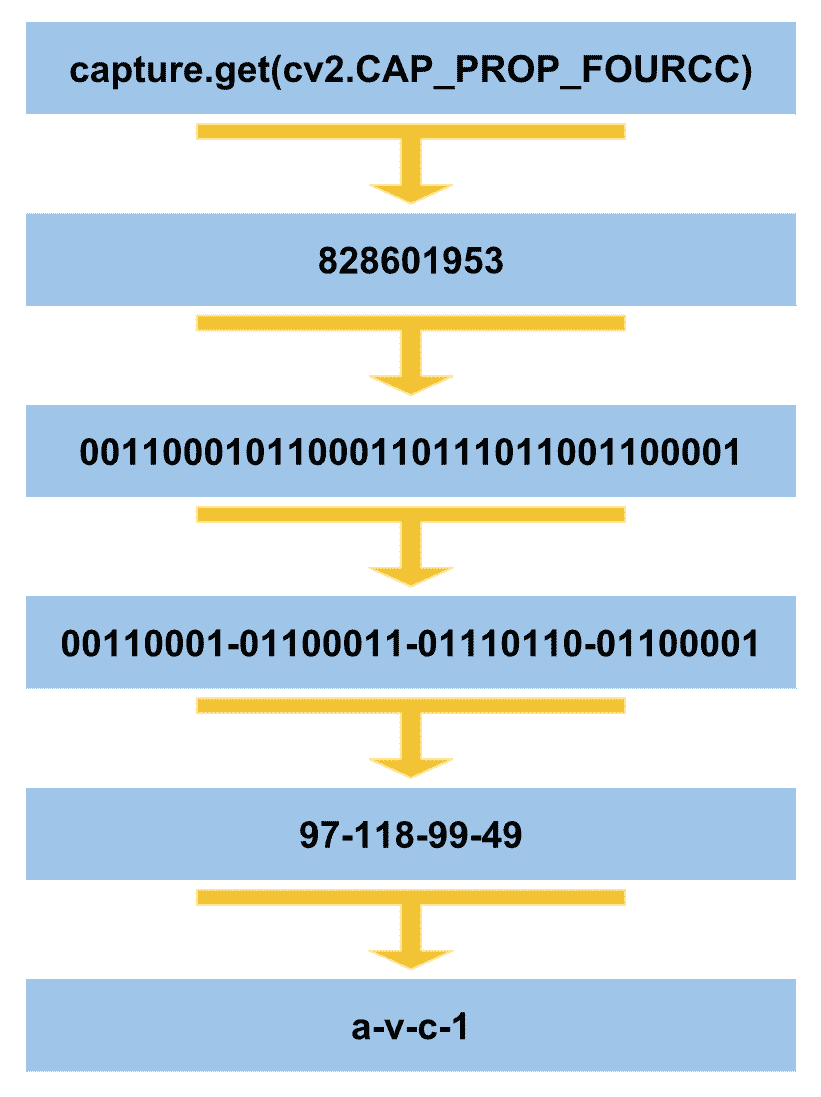# 三、处理文件和图像

• 有关处理文件和图像的理论介绍
• 读/写图像
• 读取相机帧和视频文件
• 写入视频文件
• 玩转视频捕获属性

# 技术要求

• Python 和 OpenCV
• 特定于 Python 的 IDE
• NumPy 和 Matplotlib Python 包
• Git 客户端

# 处理文件和图像的简介# sys.argv# Import the required packages
import sys

# We will print some information in connection with sys.argv to see how it works:
print("The name of the script being processed is: '{}'".format(sys.argv))
print("The number of arguments of the script is: '{}'".format(len(sys.argv)))
print("The arguments of the script are: '{}'".format(str(sys.argv)))


The name of the script being processed is: 'sysargv_python.py'
The number of arguments of the script is: '1'
The arguments of the script are: '['sysargv_python.py']'


The name of the script being processed is: 'sysargv_python.py'
The number of arguments of the script is: '2'
The arguments of the script are: '['sysargv_python.py', 'OpenCV']'


argv是脚本名，如果它不是完整路径名，则取决于操作系统。 请参阅这里了解更多信息。

# argparse – 命令行选项和参数解析

# Import the required packages
import argparse

# We first create the ArgumentParser object
# The created object 'parser' will have the necessary information
# to parse the command-line arguments into data types.
parser = argparse.ArgumentParser()

# The information about program arguments is stored in 'parser' and used when parse_args() is called.
# ArgumentParser parses arguments through the parse_args() method:
parser.parse_args()


usage: argparse_minimal.py [-h]
optional arguments:
-h, --help show this help message and exit


argparse_minimal.py 6
usage: argparse_minimal.py [-h]
argparse_minimal.py: error: unrecognized arguments: 6


# Import the required packages
import argparse

# We first create the ArgumentParser object
# The created object 'parser' will have the necessary information
# to parse the command-line arguments into data types.
parser = argparse.ArgumentParser()

parser.add_argument("first_argument", help="this is the string text in connection with first_argument")

# The information about program arguments is stored in 'parser'
# Then, it is used when the parser calls parse_args().
# ArgumentParser parses arguments through the parse_args() method:
args = parser.parse_args()

# We get and print the first argument of this script:
print(args.first_argument)


usage: argparse_positional_arguments.py [-h] first_argument
argparse_positional_arguments.py: error: the following arguments are required: first_argument


usage: argparse_positional_arguments.py [-h] first_argument
positional arguments:
first_argument this is the string text in connection with first_argument
optional arguments:
-h, --help show this help message and exit


# Import the required packages
import argparse

# We first create the ArgumentParser object
# The created object 'parser' will have the necessary information
# to parse the command-line arguments into data types.
parser = argparse.ArgumentParser()

# We add 'first_number' argument using add_argument() including a help. The type of this argument is int

# We add 'second_number' argument using add_argument() including a help The type of this argument is int

# The information about program arguments is stored in 'parser'
# Then, it is used when the parser calls parse_args().
# ArgumentParser parses arguments through the parse_args() method:
args = parser.parse_args()
print("args: '{}'".format(args))

print("the sum is: '{}'".format(args.first_number + args.second_number))

# Additionally, the arguments can be stored in a dictionary calling vars() function:
args_dict = vars(parser.parse_args())

# We print this dictionary:
print("args_dict dictionary: '{}'".format(args_dict))

# For example, to get the first argument using this dictionary:
print("first argument from the dictionary: '{}'".format(args_dict["first_number"]))


argparse_sum_two_numbers.py
usage: argparse_sum_two_numbers.py [-h] first_number second_number
argparse_sum_two_numbers.py: error: the following arguments are required: first_number, second_number


argparse_sum_two_numbers.py --help
usage: argparse_sum_two_numbers.py [-h] first_number second_number

positional arguments:
first_number first number to be added
second_number second number to be added

optional arguments:
-h, --help show this help message and exit


# Additionally, the arguments can be stored in a dictionary calling vars() function:
args_dict = vars(parser.parse_args())

# We print this dictionary:
print("args_dict dictionary: '{}'".format(args_dict))

# For example, to get the first argument using this dictionary:
print("first argument from the dictionary: '{}'".format(args_dict["first_number"]))


args: 'Namespace(first_number=5, second_number=10)'
the sum is: '15'
args_dict dictionary: '{'first_number': 5, 'second_number': 10}'
first argument from the dictionary: '5'


# 在 OpenCV 中读取图像

# Import the required packages
import argparse
import cv2

# We first create the ArgumentParser object
# The created object 'parser' will have the necessary information
# to parse the command-line arguments into data types.
parser = argparse.ArgumentParser()

# We add 'path_image' argument using add_argument() including a help. The type of this argument is string (by default)
parser.add_argument("path_image", help="path to input image to be displayed")

# The information about program arguments is stored in 'parser'
# Then, it is used when the parser calls parse_args().
# ArgumentParser parses arguments through the parse_args() method:
args = parser.parse_args()

# We can now load the input image from disk:

# Parse the argument and store it in a dictionary:
args = vars(parser.parse_args())

# Now, we can also load the input image from disk using args:

# Wait until a key is pressed:
cv2.waitKey(0)

# Destroy all windows:
cv2.destroyAllWindows()


# 在 OpenCV 中读取和写入图像

# Import the required packages
import argparse
import cv2

# We first create the ArgumentParser object
# The created object 'parser' will have the necessary information
# to parse the command-line arguments into data types.
parser = argparse.ArgumentParser()

# Add 'path_image_input' argument using add_argument() including a help. The type is string (by default):
parser.add_argument("path_image_input", help="path to input image to be displayed")

# Add 'path_image_output' argument using add_argument() including a help. The type is string (by default):
parser.add_argument("path_image_output", help="path of the processed image to be saved")

# Parse the argument and store it in a dictionary:
args = vars(parser.parse_args())

# We can load the input image from disk:

# Process the input image (convert it to grayscale):
gray_image = cv2.cvtColor(image_input, cv2.COLOR_BGR2GRAY)

# Show the processed image:
cv2.imshow("gray image", gray_image)

# Save the processed image to disk:
cv2.imwrite(args["path_image_output"], gray_image)

# Wait until a key is pressed:
cv2.waitKey(0)

# Destroy all windows:
cv2.destroyAllWindows()


# Process the input image (convert it to grayscale)
gray_image = cv2.cvtColor(image_input, cv2.COLOR_BGR2GRAY)


# 读取相机帧

 # We create a VideoCapture object to read from the camera (pass 0):
capture = cv2.VideoCapture(args.index_camera)


gray_frame = cv2.cvtColor(frame, cv2.COLOR_BGR2GRAY)


# 访问捕获对象的某些属性

# Import the required packages
import cv2
import argparse

# We first create the ArgumentParser object
# The created object 'parser' will have the necessary information
# to parse the command-line arguments into data types.
parser = argparse.ArgumentParser()

args = parser.parse_args()

# We create a VideoCapture object to read from the camera (pass 0):
capture = cv2.VideoCapture(args.index_camera)

# Get some properties of VideoCapture (frame width, frame height and frames per second (fps)):
frame_width = capture.get(cv2.CAP_PROP_FRAME_WIDTH)
frame_height = capture.get(cv2.CAP_PROP_FRAME_HEIGHT)
fps = capture.get(cv2.CAP_PROP_FPS)

# Print these values:
print("CV_CAP_PROP_FRAME_WIDTH: '{}'".format(frame_width))
print("CV_CAP_PROP_FRAME_HEIGHT : '{}'".format(frame_height))
print("CAP_PROP_FPS : '{}'".format(fps))

# Check if camera opened successfully
if capture.isOpened()is False:
print("Error opening the camera")

# Read until video is completed
while capture.isOpened():
# Capture frame-by-frame from the camera

if ret is True:
# Display the captured frame:
cv2.imshow('Input frame from the camera', frame)

# Convert the frame captured from the camera to grayscale:
gray_frame = cv2.cvtColor(frame, cv2.COLOR_BGR2GRAY)

# Display the grayscale frame:
cv2.imshow('Grayscale input camera', gray_frame)

# Press q on keyboard to exit the program
if cv2.waitKey(20) & 0xFF == ord('q'):
break
# Break the loop
else:
break

# Release everything:
capture.release()
cv2.destroyAllWindows()


# 保存相机帧

 # Press c on keyboard to save current frame
if cv2.waitKey(20) & 0xFF == ord('c'):
frame_name = "camera_frame_{}.png".format(frame_index)
gray_frame_name = "grayscale_camera_frame_{}.png".format(frame_index)
cv2.imwrite(frame_name, frame)
cv2.imwrite(gray_frame_name, gray_frame)
frame_index += 1


ord('c')使用八位返回代表c字符的值。 此外，cv2.waitKey()值是按位的，并且将&运算符与0xFF一起使用只能得到其最后八位。 因此，我们可以在这两个 8 位值之间进行比较。 当按下C键时，我们为两个帧建立名称。 然后，我们将两个图像保存到磁盘。 最后，增加frame_index，以便为保存下一帧做好准备。 请查看read_camera_capture.py以查看此脚本的完整代码。

# 读取视频文件

cv2.VideoCapture也允许我们阅读视频文件。 因此，要读取视频文件，在创建cv2.VideoCapture对象时应提供视频文件的路径：

# We first create the ArgumentParser object
# The created object 'parser' will have the necessary information
# to parse the command-line arguments into data types.
parser = argparse.ArgumentParser()

parser.add_argument("video_path", help="path to the video file")
args = parser.parse_args()

# Create a VideoCapture object. In this case, the argument is the video file name:
capture = cv2.VideoCapture(args.video_path)


# 从 IP 摄像机读取# 计算每秒帧

# Read until the video is completed, or 'q' is pressed
while capture.isOpened():
# Capture frame-by-frame from the camera

if ret is True:
# Calculate time before processing the frame:
processing_start = time.time()

# All the processing should be included here
# ...
# ...
# End of processing

# Calculate time after processing the frame
processing_end = time.time()

# Calculate the difference
processing_time_frame = processing_end - processing_start

# FPS = 1 / time_per_frame
# Show the number of frames per second
print("fps: {}".format(1.0 / processing_time_frame))

# Break the loop
else:
break



processing_start = time.time()


processing_end = time.time()


processing_time_frame = processing_end - processing_start


print("fps: {}".format(1.0 / processing_time_frame))


# 写入视频文件的注意事项# We first create the ArgumentParser object
# The created object 'parser' will have the necessary information
# to parse the command-line arguments into data types.
parser = argparse.ArgumentParser()

parser.add_argument("output_video_path", help="path to the video file to write")
args = parser.parse_args()


# Create a VideoCapture object and pass 0 as argument to read from the camera
capture = cv2.VideoCapture(0)


# Get some properties of VideoCapture (frame width, frame height and frames per second (fps)):
frame_width = capture.get(cv2.CAP_PROP_FRAME_WIDTH)
frame_height = capture.get(cv2.CAP_PROP_FRAME_HEIGHT)
fps = capture.get(cv2.CAP_PROP_FPS)


# FourCC is a 4-byte code used to specify the video codec and it is platform dependent!
# In this case, define the codec XVID
fourcc = cv2.VideoWriter_fourcc('X', 'V', 'I', 'D')


# FourCC is a 4-byte code used to specify the video codec and it is platform dependent!
# In this case, define the codec XVID
fourcc = cv2.VideoWriter_fourcc(*'XVID')


# Create VideoWriter object. We use the same properties as the input camera.
# Last argument is False to write the video in grayscale. True otherwise (write the video in color)
out_gray = cv2.VideoWriter(args.output_video_path, fourcc, int(fps), (int(frame_width), int(frame_height)), False)


# Read until video is completed or 'q' is pressed
while capture.isOpened():
# Read the frame from the camera
if ret is True:

# Convert the frame to grayscale
gray_frame = cv2.cvtColor(frame, cv2.COLOR_BGR2GRAY)

# Write the grayscale frame to the video
out_gray.write(gray_frame)

# We show the frame (this is not necessary to write the video)
# But we show it until 'q' is pressed
cv2.imshow('gray', gray_frame)
if cv2.waitKey(1) & 0xFF == ord('q'):
break
else:
break


# Release everything:
capture.release()
out_gray.release()
cv2.destroyAllWindows()


# 从视频捕获对象获取所有属性

def decode_fourcc(fourcc):
"""Decodes the fourcc value to get the four chars identifying it

"""
fourcc_int = int(fourcc)

# We print the int value of fourcc
print("int value of fourcc: '{}'".format(fourcc_int))

# We can also perform this in one line:
# return "".join([chr((fourcc_int >> 8 * i) & 0xFF) for i in range(4)])

fourcc_decode = ""
for i in range(4):
int_value = fourcc_int >> 8 * i & 0xFF
print("int_value: '{}'".format(int_value))
fourcc_decode += chr(int_value)
return fourcc_decodereturn "".join([chr((fourcc_int >> 8 * i) & 0xFF) for i in range(4)])


CAP_PROP_POS_FRAMES属性为您提供视频文件的当前帧，CAP_PROP_POS_MSEC属性为您提供当前帧的时间戳。 我们还可以使用CAP_PROP_FPS属性获取 fps 的数量。 CAP_PROP_FRAME_COUNT属性为您提供视频文件的总帧数。

# Get and print these values:
print("CV_CAP_PROP_FRAME_WIDTH: '{}'".format(capture.get(cv2.CAP_PROP_FRAME_WIDTH)))
print("CV_CAP_PROP_FRAME_HEIGHT : '{}'".format(capture.get(cv2.CAP_PROP_FRAME_HEIGHT)))
print("CAP_PROP_FPS : '{}'".format(capture.get(cv2.CAP_PROP_FPS)))
print("CAP_PROP_POS_MSEC : '{}'".format(capture.get(cv2.CAP_PROP_POS_MSEC)))
print("CAP_PROP_POS_FRAMES : '{}'".format(capture.get(cv2.CAP_PROP_POS_FRAMES)))
print("CAP_PROP_FOURCC  : '{}'".format(decode_fourcc(capture.get(cv2.CAP_PROP_FOURCC))))
print("CAP_PROP_FRAME_COUNT  : '{}'".format(capture.get(cv2.CAP_PROP_FRAME_COUNT)))
print("CAP_PROP_MODE : '{}'".format(capture.get(cv2.CAP_PROP_MODE)))
print("CAP_PROP_BRIGHTNESS : '{}'".format(capture.get(cv2.CAP_PROP_BRIGHTNESS)))
print("CAP_PROP_CONTRAST : '{}'".format(capture.get(cv2.CAP_PROP_CONTRAST)))
print("CAP_PROP_SATURATION : '{}'".format(capture.get(cv2.CAP_PROP_SATURATION)))
print("CAP_PROP_HUE : '{}'".format(capture.get(cv2.CAP_PROP_HUE)))
print("CAP_PROP_GAIN  : '{}'".format(capture.get(cv2.CAP_PROP_GAIN)))
print("CAP_PROP_EXPOSURE : '{}'".format(capture.get(cv2.CAP_PROP_EXPOSURE)))
print("CAP_PROP_CONVERT_RGB : '{}'".format(capture.get(cv2.CAP_PROP_CONVERT_RGB)))
print("CAP_PROP_RECTIFICATION : '{}'".format(capture.get(cv2.CAP_PROP_RECTIFICATION)))
print("CAP_PROP_ISO_SPEED : '{}'".format(capture.get(cv2.CAP_PROP_ISO_SPEED)))
print("CAP_PROP_BUFFERSIZE : '{}'".format(capture.get(cv2.CAP_PROP_BUFFERSIZE)))


# 使用属性来反向播放视频

• cv2.CAP_PROP_FRAME_COUNT：此属性提供帧的总数
• cv2.CAP_PROP_POS_FRAMES：此属性提供当前帧

# We get the index of the last frame of the video file
frame_index = capture.get(cv2.CAP_PROP_FRAME_COUNT) - 1


 # We set the current frame position
capture.set(cv2.CAP_PROP_POS_FRAMES, frame_index)


 # Capture frame-by-frame from the video file


 # Decrement the index to read next frame
frame_index = frame_index - 1


# 总结

• 显示算法的一些中间结果（例如，检测到的对象的边界框）
• 显示算法的最终结果（例如，检测到的对象的类别，例如汽车，猫或狗）
• 显示一些调试信息（例如，执行时间）

# 问题

1. 什么是sys.argv
2. 编写一段代码以添加int类型的first_number参数，并包括要使用parser.add_argument()添加的帮助优先编号。
3. 编写一段代码，将想象中的img保存到名称为image.png的磁盘上。
4. 使用cv2.VideoCapture()创建capture对象，以从连接到计算机的第一台摄像机读取。
5. 使用cv2.VideoCapture()创建对象捕获，以从连接到计算机的第一台摄像机读取并打印CAP_PROP_FRAME_WIDTH属性。
6. 读取图像并将其保存到名称相同但以_copy.png结尾的磁盘（例如logo_copy.png）。
7. 创建一个脚本（read_video_file_backwards_save_video.py），以加载视频文件并创建另一个向后播放的脚本（首先包含视频的最后一帧，依此类推）。